========================================================

Earlier you experimented with some basic regression analysis and during the lecture the idea of ‘geographically weighted regression’ (GWR) was introduced. In regression analysis you can take a dependent variable (in our case Average GCSE Scores in Wards across London) and try and explain variation these scores using an independent variable (such as level of unauthorised school absence) or a suite of uncorrelated and normally distributed independent variables. The strength and direction of association is indicated by the regression coefficients, with one coefficient given for each variable in the dataset. In GWR, instead of one global coefficient for each variable, coefficients are able to vary according to space. This spatial variation in coefficients can reveal interesting patterns which otherwise would be masked.

This practical exercise will only skirt the practical edges of GWR, for much more detail you should visit the GWR website which is produced and maintained by the people that originally developed the technique - http://gwr.nuim.ie/

There are various packages which will carry out GWR in R, for this pracical we we use spgwr (mainly because it was the first one I came across), although you could also use GWmodel or gwrr. At the end of this practical, you can test out these ideas in ArcGIS using the GWR toolset in the Spatial Statistics Toolbox - http://resources.arcgis.com/en/help/main/10.1/index.html#/Geographically_Weighted_Regression_GWR/005p00000021000000/

I should also acknowledge the guide on GWR (accessible here: http://www.bris.ac.uk/cmpo/events/2009/segregation/gwr.pdf) produced by the University of Bristol, which was a great help when producing this exercise.

## Getting Started

First we need to set our working directory and library the packages we will be using:

library(spgwr)
## Loading required package: sp
## NOTE: This package does not constitute approval of GWR
## as a method of spatial analysis; see example(gwr)
library(ggplot2)
library(maptools)
## Checking rgeos availability: TRUE
setwd("Z:/GIS/wk7")
attach(LondonWards)

The first thing I would like you to do is run the multiple regression model you created earlier in the practical - below is just one example of such a model:

model1 <- lm(AvgGCSE2011 ~ UnauthAbsenceSchools11+PctWithNoQual11+CarsPerHH2011)
summary(model1)
##
## Call:
## lm(formula = AvgGCSE2011 ~ UnauthAbsenceSchools11 + PctWithNoQual11 +
##     CarsPerHH2011)
##
## Residuals:
##     Min      1Q  Median      3Q     Max
## -116.86   -8.08    0.40    8.38   45.41
##
## Coefficients:
##                        Estimate Std. Error t value Pr(>|t|)
## (Intercept)             361.891      3.071  117.84  < 2e-16 ***
## UnauthAbsenceSchools11  -13.008      1.870   -6.96  8.9e-12 ***
## PctWithNoQual11          -1.194      0.107  -11.19  < 2e-16 ***
## CarsPerHH2011            23.355      2.147   10.88  < 2e-16 ***
## ---
## Signif. codes:  0 '***' 0.001 '**' 0.01 '*' 0.05 '.' 0.1 ' ' 1
##
## Residual standard error: 13.6 on 621 degrees of freedom
## Multiple R-squared:  0.516,  Adjusted R-squared:  0.514
## F-statistic:  221 on 3 and 621 DF,  p-value: <2e-16

In this example you can see that we have a significant model (p<0.01), with an R-squared of around 52% and negative relationships between unauthorised absence from school and percent of the population with no qualifications and average GCSE scores in 2011, and a positive relationship between the average number of cars per household and average GCSE scores in 2011.

Checking the catch-all plot associated with this model (standardised residuals plotted agains the fitted values), we can see that as there is no descernable pattern in the cloud of points, our model is correctly specified.

plot(model1, which=3)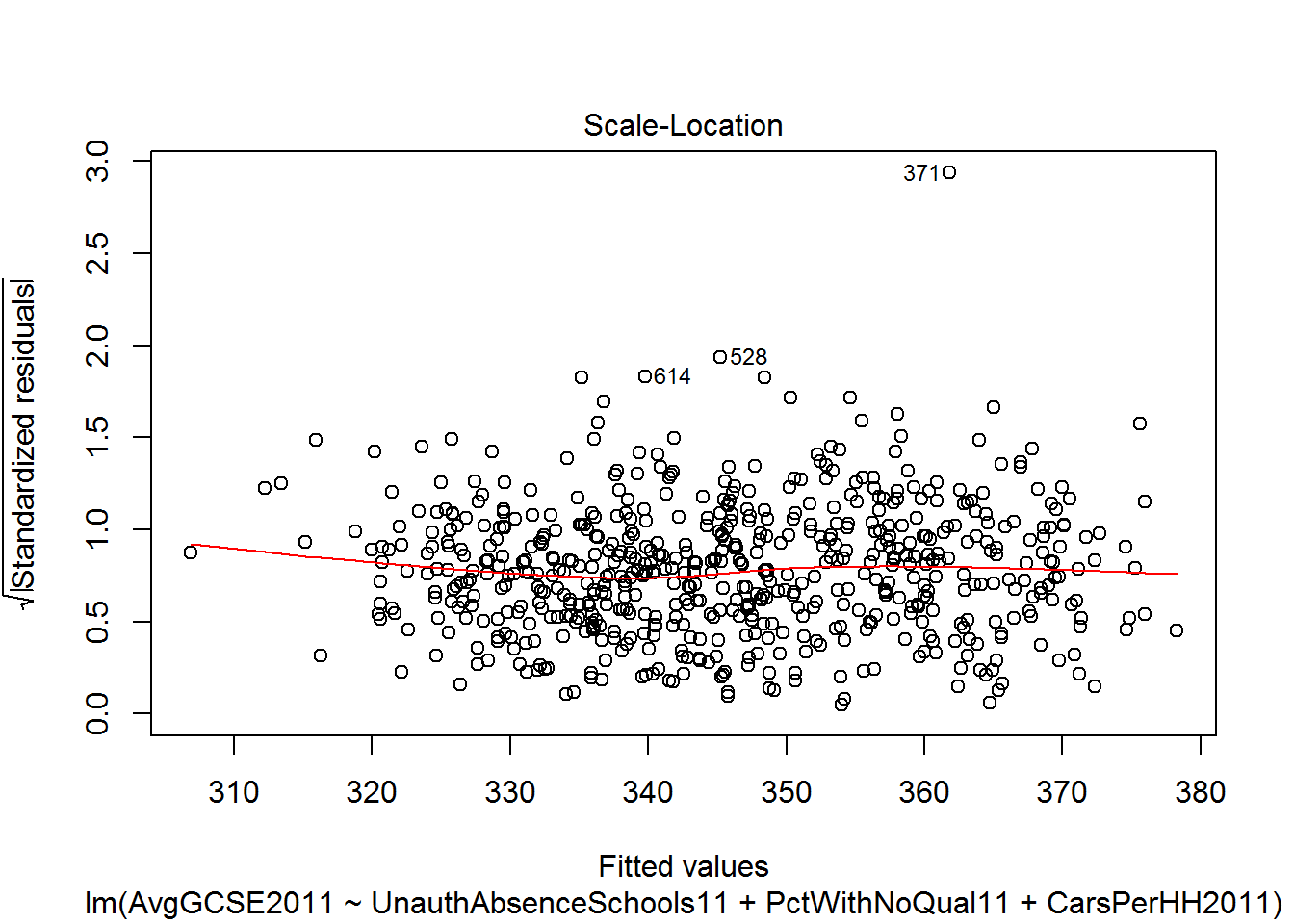One of the things we can now do is plot the residuals to see if there is any obvious spatial patterning

resids<-residuals(model1)
colours <- c("dark blue", "blue", "red", "dark red")
#here it is assumed that your eastings and northings coordinates are stored in columns called x and y in your dataframe
map.resids <- SpatialPointsDataFrame(data=data.frame(resids), coords=cbind(x,y))
#for speed we are just going to use the quick sp plot function, but you could alternatively store your residuals back in your LondonWards dataframe and plot using geom_point in ggplot2
spplot(map.resids, cuts=quantile(resids), col.regions=colours, cex=1)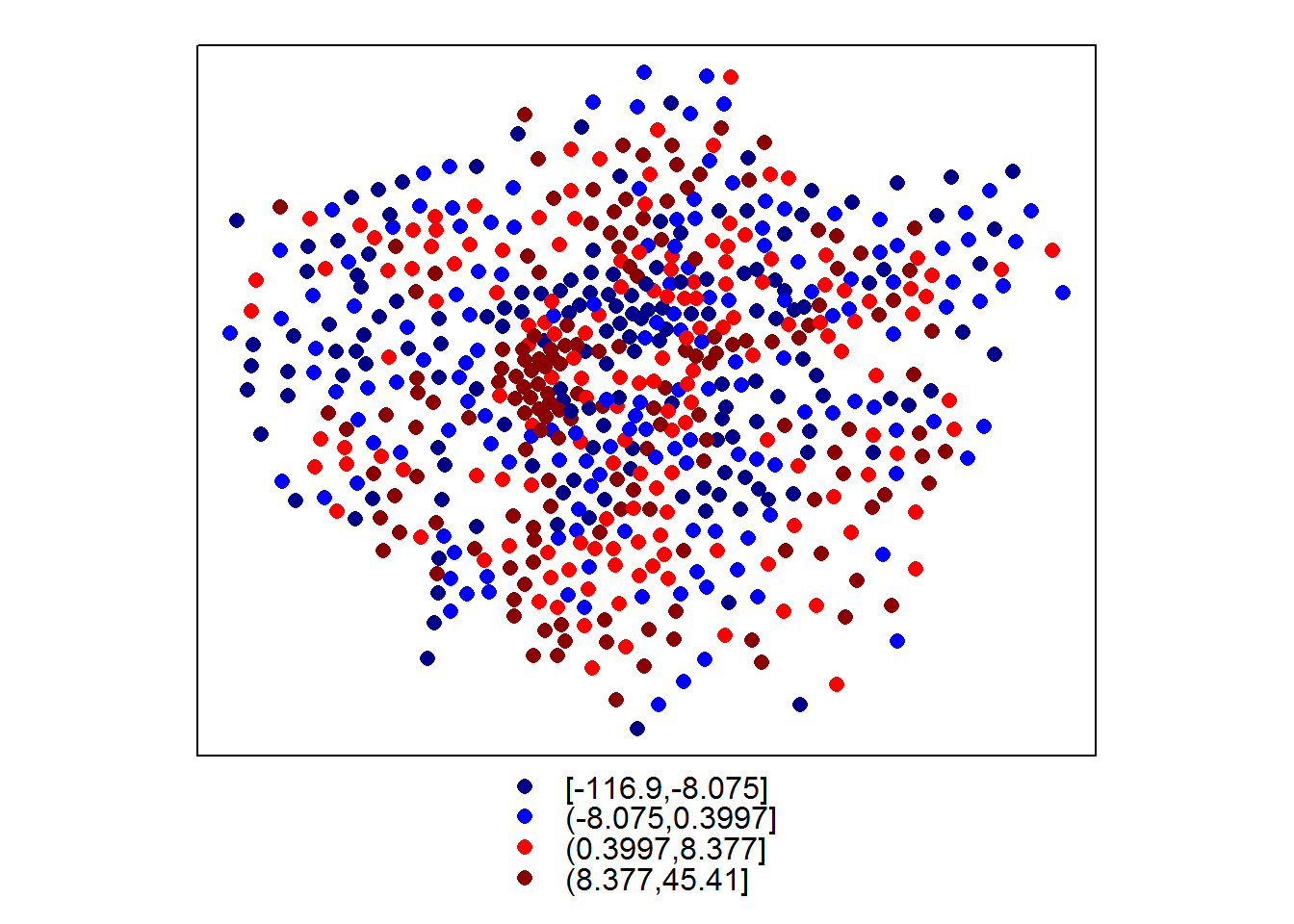From this plot it is apparent that there is some spatial patterning of the residuals (i.e. the red and blue points are not randomly distributed, but there appear to be small clusters of red and blue points in certain parts of London). As there appears to be some spatial patterning in these residuals, we will now run a geographically weighted regression model to see how the coefficients of the model might vary across London.

First we will calibrate the bandwidth of the kernel that will be used to capture the points for each regression (this may take a little while) and then run the model:

#calculate kernel bandwidth
GWRbandwidth <- gwr.sel(AvgGCSE2011 ~ UnauthAbsenceSchools11+PctWithNoQual11+CarsPerHH2011, data=LondonWards, coords=cbind(x,y),adapt=T) 
## Adaptive q: 0.382 CV score: 114291
## Adaptive q: 0.618 CV score: 115394
## Adaptive q: 0.2361 CV score: 112803
## Adaptive q: 0.1459 CV score: 110782
## Adaptive q: 0.09017 CV score: 108582
## Adaptive q: 0.05573 CV score: 106917
## Adaptive q: 0.03444 CV score: 105735
## Adaptive q: 0.02129 CV score: 105044
## Adaptive q: 0.01316 CV score: 105155
## Adaptive q: 0.01941 CV score: 105027
## Adaptive q: 0.01909 CV score: 105011
## Adaptive q: 0.01682 CV score: 104690
## Adaptive q: 0.01542 CV score: 104756
## Adaptive q: 0.01658 CV score: 104684
## Adaptive q: 0.01649 CV score: 104681
## Adaptive q: 0.01608 CV score: 104674
## Adaptive q: 0.0156 CV score: 104729
## Adaptive q: 0.0159 CV score: 104687
## Adaptive q: 0.01624 CV score: 104676
## Adaptive q: 0.01601 CV score: 104674
## Adaptive q: 0.01597 CV score: 104678
## Adaptive q: 0.01601 CV score: 104674
#run the gwr model
gwr.model = gwr(AvgGCSE2011 ~ UnauthAbsenceSchools11+PctWithNoQual11+CarsPerHH2011, data=LondonWards, coords=cbind(x,y), adapt=GWRbandwidth, hatmatrix=TRUE, se.fit=TRUE)
#print the results of the model
gwr.model
## Call:
## gwr(formula = AvgGCSE2011 ~ UnauthAbsenceSchools11 + PctWithNoQual11 +
##     CarsPerHH2011, data = LondonWards, coords = cbind(x, y),
##     adapt = GWRbandwidth, hatmatrix = TRUE, se.fit = TRUE)
## Kernel function: gwr.Gauss
## Summary of GWR coefficient estimates at data points:
##                           Min. 1st Qu.  Median 3rd Qu.    Max. Global
## X.Intercept.           308.000 347.000 359.000 372.000 418.000 361.89
## UnauthAbsenceSchools11 -38.500 -17.400 -13.200  -7.550  25.000 -13.01
## PctWithNoQual11         -2.560  -1.670  -1.160  -0.492   0.556  -1.19
## CarsPerHH2011          -38.000  13.300  23.800  33.500  67.400  23.35
## Number of data points: 625
## Effective number of parameters (residual: 2traceS - traceS'S): 125.8
## Effective degrees of freedom (residual: 2traceS - traceS'S): 499.2
## Sigma (residual: 2traceS - traceS'S): 12.26
## Effective number of parameters (model: traceS): 89.11
## Effective degrees of freedom (model: traceS): 535.9
## Sigma (model: traceS): 11.83
## Sigma (ML): 10.95
## AICc (GWR p. 61, eq 2.33; p. 96, eq. 4.21): 4977
## AIC (GWR p. 96, eq. 4.22): 4855
## Residual sum of squares: 74999
## Quasi-global R2: 0.6852

The output from the GWR model reveals how the coefficients vary across the 625 Wards in our London Study region. You will see how the global coefficients are exactly the same as the coefficients in the earlier lm model. In this particular model (yours will look a little different if you have used different explanatory variables), if we take unauthorised absence from school, we can see that the coefficients range from a minimum value of -38.54 (1 unit change in unauthorised absence resulting in a drop in average GCSE score of -38.54) to +25.05 (1 unit change in unauthorised absence resulting in an increase in average GCSE score of +25.05). For half of the wards in the dataset, as unauthorised absence rises by 1 point, GCSE scores will decrease between -17.43 and -7.55 points (the inter-quartile range between the 1st Qu and the 3rd Qu).

Coefficient ranges can also be seen for the other variables and they suggest some interesting spatial patterning. To explore this we can plot the GWR coefficients for different variables. Firstly we can attach the coefficients to our original dataframe - this can be achieved simply as the coefficients for each ward appear in the same order in our spatial points dataframe as they do in the original dataframe.

results<-as.data.frame(gwr.model$SDF) head(results) ## sum.w X.Intercept. UnauthAbsenceSchools11 PctWithNoQual11 CarsPerHH2011 ## 1 22.48 359.4 -27.56 -0.4589 40.506 ## 2 16.19 351.2 -15.58 -0.2908 18.204 ## 3 15.53 364.2 -12.10 -0.6325 4.284 ## 4 16.11 364.8 -18.18 -0.4293 8.543 ## 5 20.00 375.0 -13.45 -1.0741 7.107 ## 6 16.33 373.1 -14.32 -0.8786 4.399 ## X.Intercept._se UnauthAbsenceSchools11_se PctWithNoQual11_se ## 1 12.68 7.259 0.3830 ## 2 20.20 8.123 0.4784 ## 3 24.89 10.420 0.4149 ## 4 22.73 10.475 0.4168 ## 5 19.08 8.304 0.3597 ## 6 25.17 9.946 0.4483 ## CarsPerHH2011_se gwr.e pred pred.se localR2 X.Intercept._se_EDF ## 1 20.10 3.174 359.9 6.320 0.4984 13.13 ## 2 12.24 1.930 333.4 4.399 0.4875 20.93 ## 3 14.88 2.706 330.4 3.545 0.4490 25.79 ## 4 13.37 -5.793 332.8 3.427 0.4885 23.55 ## 5 10.98 10.216 329.6 3.314 0.6399 19.77 ## 6 14.95 -6.855 336.4 2.914 0.6324 26.08 ## UnauthAbsenceSchools11_se_EDF PctWithNoQual11_se_EDF ## 1 7.520 0.3968 ## 2 8.416 0.4957 ## 3 10.796 0.4299 ## 4 10.853 0.4318 ## 5 8.603 0.3727 ## 6 10.304 0.4645 ## CarsPerHH2011_se_EDF pred.se_EDF x y ## 1 20.83 6.548 532480 181272 ## 2 12.68 4.557 544218 184361 ## 3 15.42 3.673 549062 185153 ## 4 13.85 3.551 546998 186089 ## 5 11.38 3.434 548359 189492 ## 6 15.49 3.019 550789 186098 #attach coefficients to original dataframe LondonWards$coefUnauthAbsenceSchools11<-results$UnauthAbsenceSchools11 LondonWards$coefPctWithNoQual11<-results$PctWithNoQual11 LondonWards$coefCarsPerHH2011<-results$CarsPerHH2011 Now read in some borough outlines to contextualise your ward centroid points #read in the shapefile using the maptools function readShapePoly boroughs <- readShapePoly("london_boroughs.shp") #fortify for use in ggpplot2 boroughoutline <- fortify(boroughs, region="name") ## Loading required package: rgeos ## rgeos version: 0.3-4, (SVN revision 438) ## GEOS runtime version: 3.4.2-CAPI-1.8.2 r3921 ## Polygon checking: TRUE And plot the coefficients for the different variables: #now plot the various GWR coefficients gwr.point1<-ggplot(LondonWards, aes(x=x,y=y))+geom_point(aes(colour=LondonWards$coefUnauthAbsenceSchools11))+scale_colour_gradient2(low = "red", mid = "white", high = "blue", midpoint = 0, space = "rgb", na.value = "grey50", guide = "colourbar", guide_legend(title="Coefs"))
gwr.point1+geom_path(data=boroughoutline,aes(long, lat, group=id), colour="grey")+coord_equal()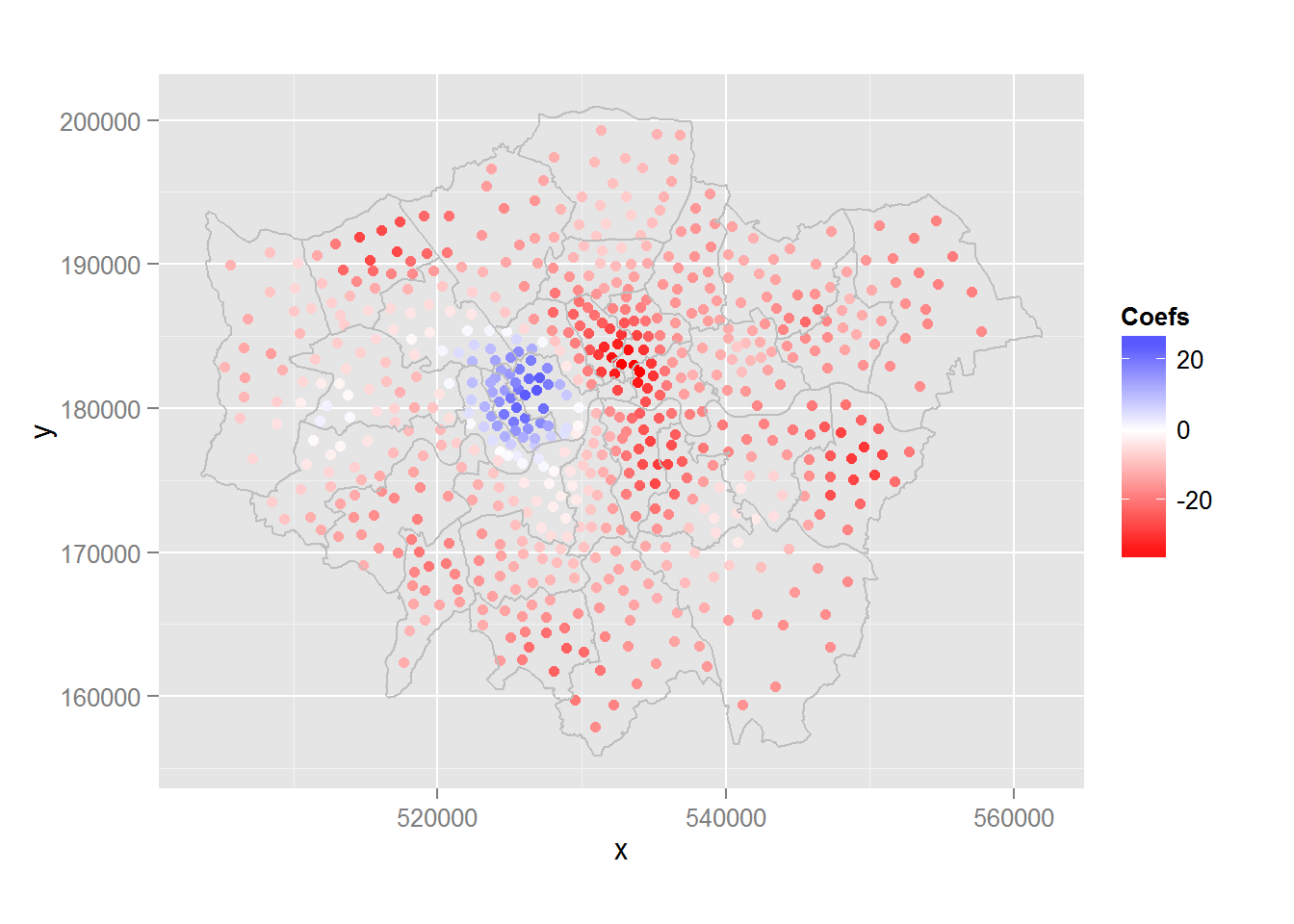gwr.point2<-ggplot(LondonWards, aes(x=x,y=y))+geom_point(aes(colour=LondonWards$coefPctWithNoQual11))+scale_colour_gradient2(low = "red", mid = "white", high = "blue", midpoint = 0, space = "rgb", na.value = "grey50", guide = "colourbar", guide_legend(title="Coefs")) gwr.point2+geom_path(data=boroughoutline,aes(long, lat, group=id), colour="grey")+coord_equal()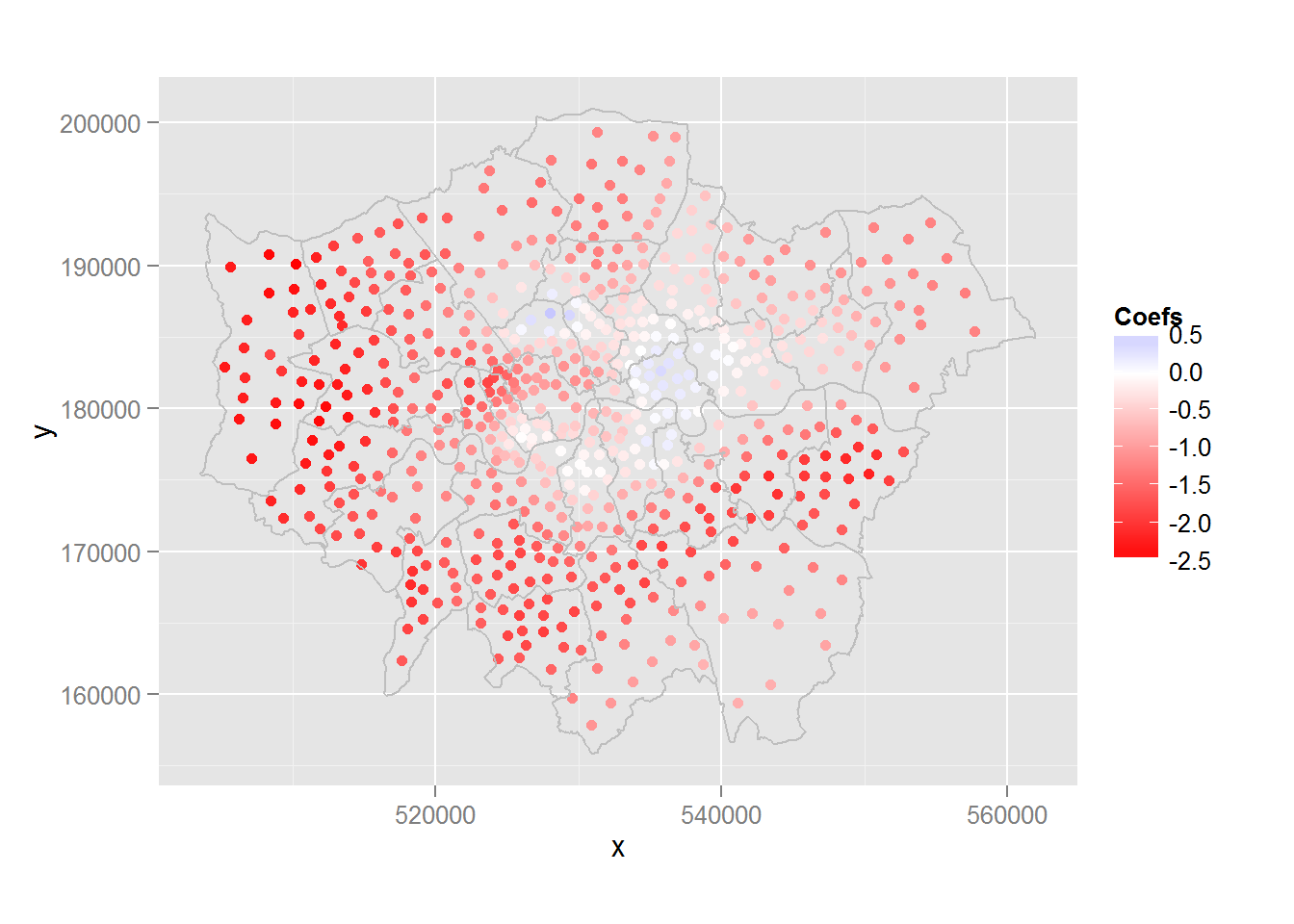gwr.point3<-ggplot(LondonWards, aes(x=x,y=y))+geom_point(aes(colour=LondonWards$coefCarsPerHH2011))+scale_colour_gradient2(low = "red", mid = "white", high = "blue", midpoint = 0, space = "rgb", na.value = "grey50", guide = "colourbar", guide_legend(title="Coefs"))
gwr.point3+geom_path(data=boroughoutline,aes(long, lat, group=id), colour="grey")+coord_equal()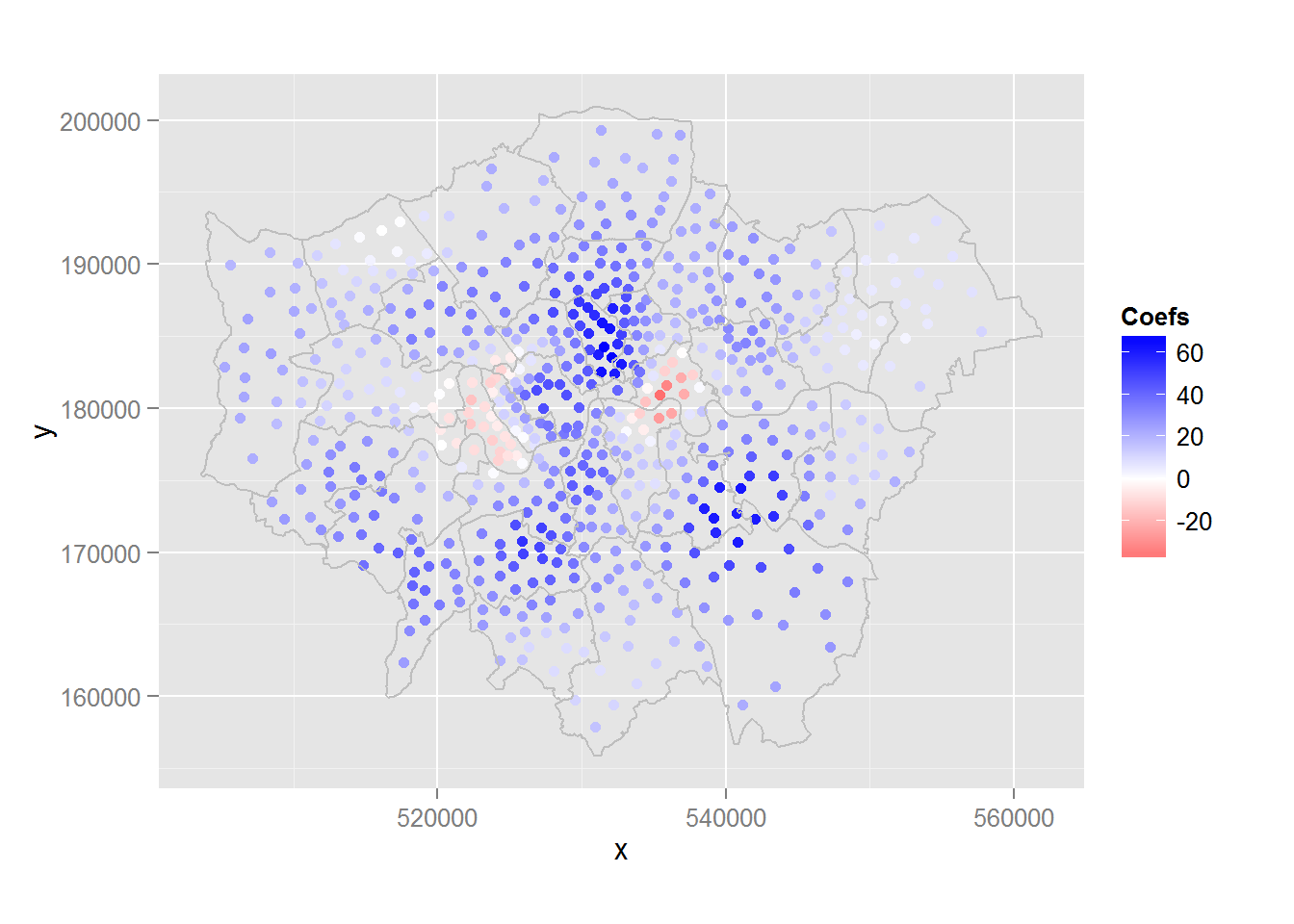Taking the first plot, which is for the unauthorised absence coefficients, we can see that for the majority of boroughs in London, there is the negative relationship we would expect - i.e. as unauthorised absence goes up, exam scores go down. For three boroughs (Westminster, Kensington & Chelsea and Hammersmith and Fulham - the richest in London), however, the relationship is positive - as unauthorised school absence increases, so does average GCSE score. This is a very interesting pattern and counterintuitive pattern, but may partly be explained the multiple homes owned by many living in these boroughs (students living in different parts of the country and indeed the world for significant periods of the year) and the over representation of private schooling of those living in these areas. If this is not the case and unauthorised absence from school is reflecting the unauthorised absence of poorer students attending local, inner city schools, then high GCSE grades may also reflect the achievements of those who are sent away to expensive fee-paying schools elsewhere in the country and who return to their parental homes later in the year. Either way, these factors could explain these results.

Of course, these results may not be statistically significant across the whole of London. Roughly speaking, if a coefficient estimate is more than 2 standard errors away from zero, then it is “statistically significant”.

To calculate standard errors, for each variable you can use a formula similar to this:

sigTest = abs(gwr.model$SDF$UnauthAbsenceSchools11) -2 * gwr.model$SDF$UnauthAbsenceSchools11_se

LondonWards\$GWRUnauthSig<-sigTest

If this is greater than zero (i.e. the estimate is more than two standard errors away from zero), it is very unlikely that the true value is zero, i.e. it is statistically significantly (at nearly the 95% confidence level)

You should now calculate these for each variable in your GWR model and See if you can plot them on a map.

From the results of your GWR exercise, what are you able to conclude about the geographical variation in your explanatory variables when predicting your dependent variable?

## ArcGIS

As mentioned at the beginning of this exercise, GWR can also be carried out using the Spatial Statistics Toolbox in ArcGIS. Using the guide in the ArcGIS help system below, try and repeat your GWR analysis using the tools available in Arc - http://resources.arcgis.com/en/help/main/10.1/index.html#/Geographically_Weighted_Regression_GWR/005p00000021000000/